Home | ARTS | Financial Management | Problems And Key - Evaluation Of Capital Projects

# Problems And Key - Evaluation Of Capital Projects

Posted On :  19.06.2018 11:53 pm

Equipment A has a cost of ` 75,000 and net cash flow of `20,000 per year for six years.

Problems And Key

1) Equipment A has a cost of ` 75,000 and net cash flow of `20,000 per year for six years. A substitute equipment B would cost ` 50,000 and generate net cash flow of ` 14,000 per year for six years. The required rate of return of both equipments is 11 per cent. Calculate the IRR and NPV for the equipments. Which equipment should be accepted and why?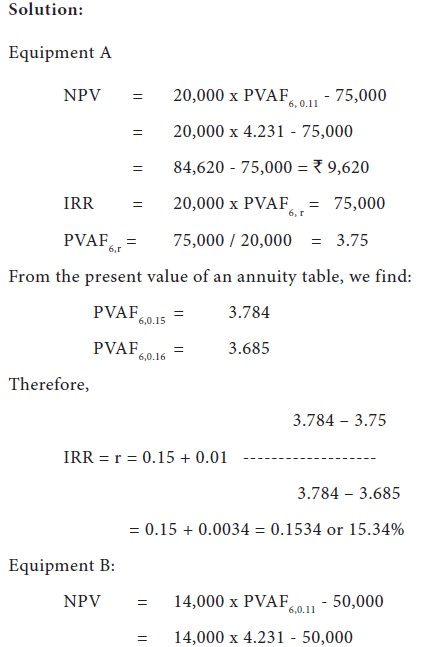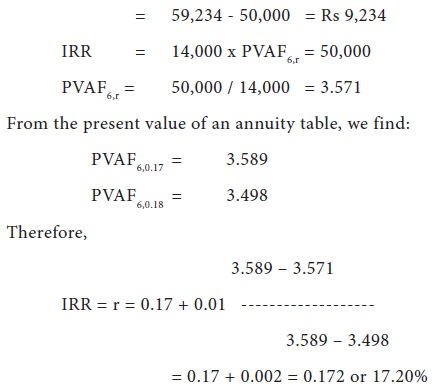Equipment A has a higher NPV but lower IRR as compared with equipment B. Therefore equipment A should be preferred since the wealth of the shareholders will be maximized.

5) For each of the following projects compute (i) pay-back period, (ii) post pay-back profitability and (iii) post-back profitability index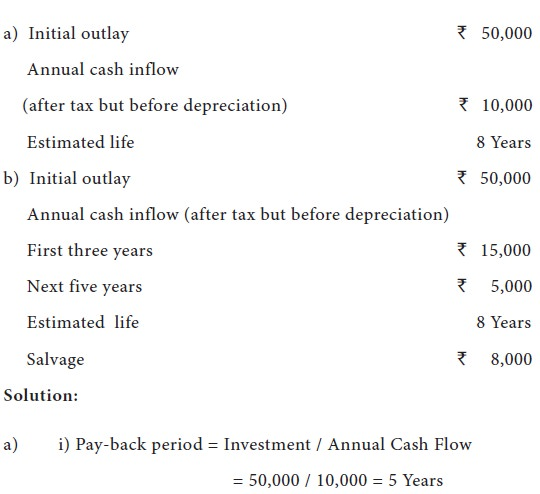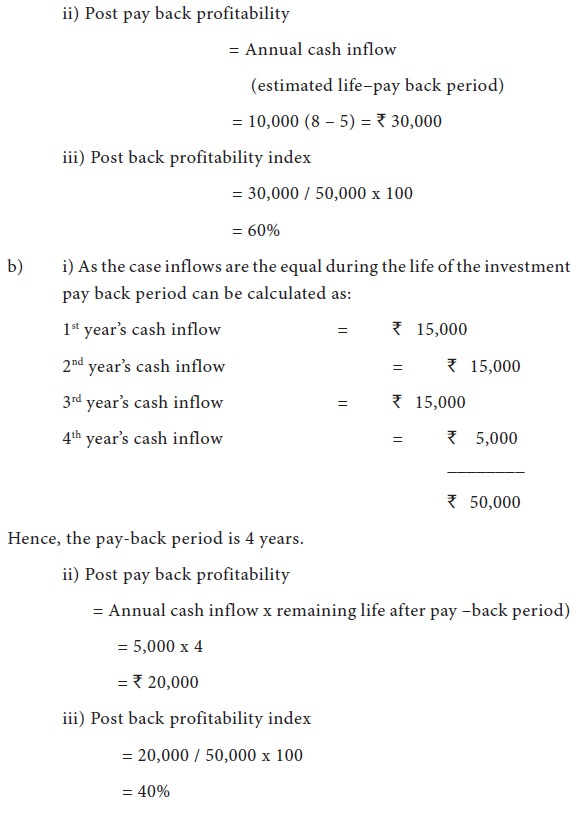6) X Ltd. is considering the purchase of a machine. Two machines are available E and F. the cost of each machine is `60,000. Each machine has expected life of 5 years. Net profits before tax and after depreciation during expected life of the machines are given below: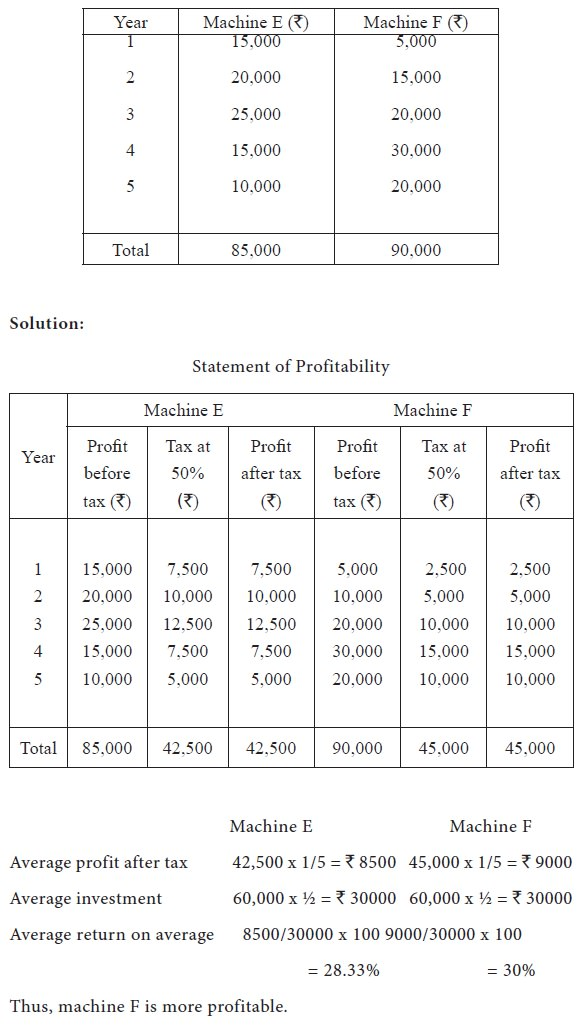7) From the following information calculate the net present value of the two projects and suggest which of the two projects should be accepted assuming a discount rate of 10%.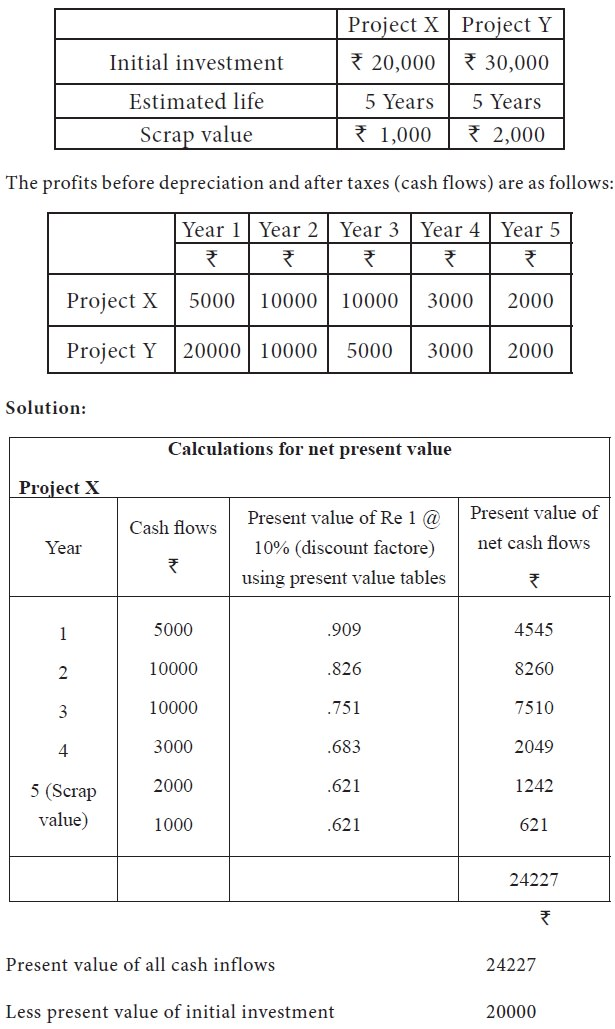(Because all the investment is to be made in the first year only, the present value is the same as the cost of the initial investment)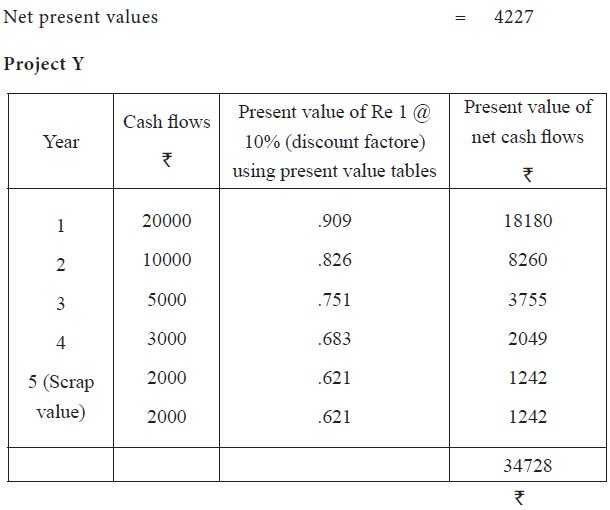Present value of all cash inflows            34728
Less present value of initial investment    30000

(Because all the investment is to be made in the first year only, the present value is the same as the cost of the initial investment)

Net present values = 4728

We find that net present value of Project Y is higher than that the net present value of Project X and hence it is suggested that project Y should be selected.

9) Two mutually exclusive investment proposals are being considered. The following information is available.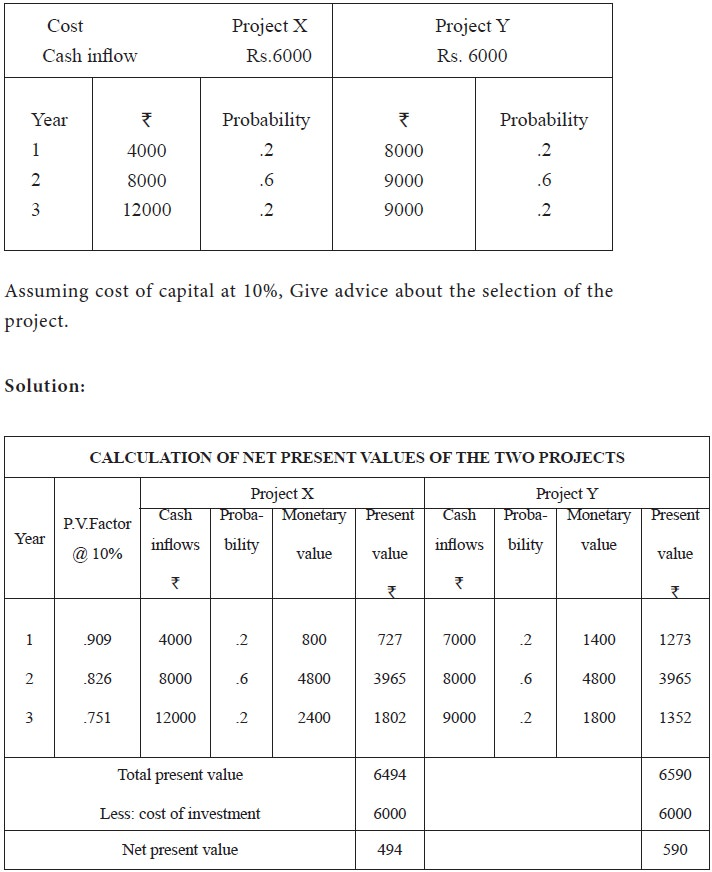As net present value of project is more than that of project X after taking into consideration the probabilities of cash inflows. Project Y is more profitable.
Tags : Financial Management - Capital Budgeting – A Conceptual Framework
Last 30 days 107 views